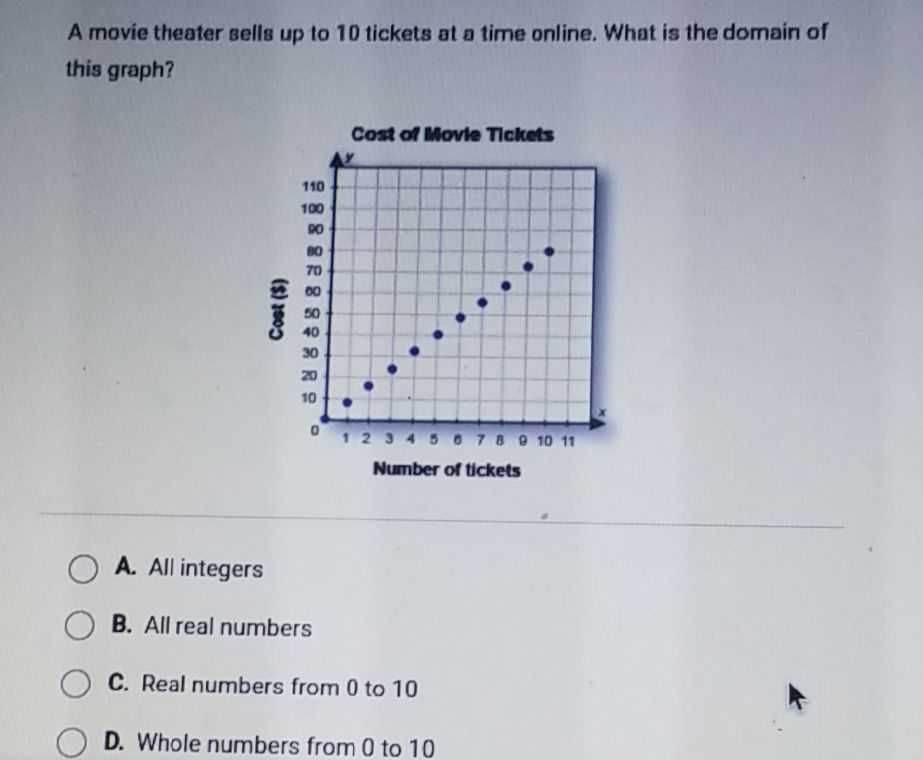### ¿Todavía tienes preguntas de matemáticas?

Pregunte a nuestros tutores expertos
Algebra
PreguntaA movie theater sells up to $$10$$ tickets at a time online. What is the domain of this graph?

A. All integers

B. All real numbers

C. Real numbers from $$0$$ to $$10$$

D. Whole numbers from $$0$$ to $$10$$